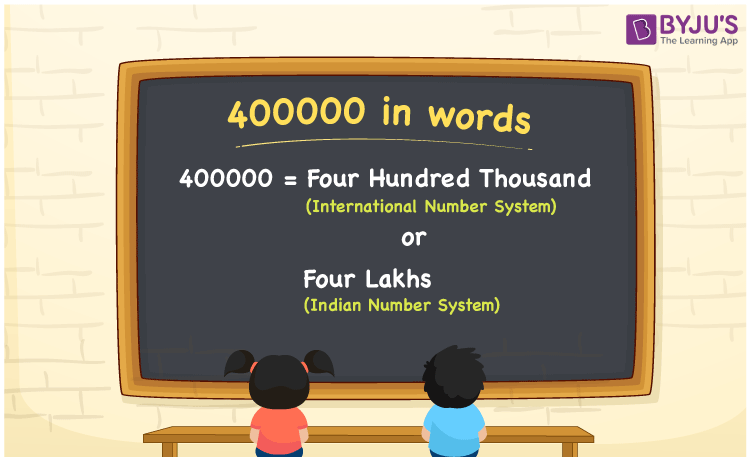# 400000 in Words

In English words, the number 400000 is expressed as “Four Hundred Thousand” or “Four Lakhs”. The number name 400000 is written using place values such as ones, tens, and hundreds. As a result, while writing the number 400000 in English, the place value table helps a lot. For example, if you purchased clothes for Rs. 400000, you can write, “I purchased clothes for Rs. Four hundred thousand”. Here, we will look at how to spell 400000 in English words as well as how to represent the number 400000 in words in detail.

 400000 in Words (International Number System): Four Hundred Thousand 400000 in Words (Indian Number System): Four Lakhs Four Hundred Thousand in Numerical Form: 400000

## 400000 in English Words## How to Write 400000 in Words?

While writing 400000 in Words, find the place value of each digit in the given number. The place value chart for the number 400000 is shown below:

 Lakhs Ten – Thousands Thousands Hundreds Tens Ones 4 0 0 0 0 0

Hence, the number 400000’s extended form is:

= 4 × Lakh + 0 × Ten thousand + 0 × Thousand + 0 × Hundred + 0 × Ten + 0 × One

= 4 × 100000 + 0 + 0 + 0 + 0 + 0

= 400000

= Four hundred thousand

Hence, 400000 in words is Four hundred thousand.

The natural number 400000 comes after 399999 but before 400001.

400000 in words – Four hundred thousand (or) Four Lakhs

Is 400000 an odd number? – No

Is 400000 an even number? – Yes

Is 400000 a perfect square number? – No

Is 400000 a perfect cube number? – No

Is 400000 a prime number? – No

Is 400000 a composite number? – Yes

## Frequently Asked Questions on 400000 in Words

### Write 400000 in words as per International Number System.

According to the International Number System, 400000 in words is four hundred thousand.

### Write 400000 in words as per the Indian Number System.

According to the Indian Number System, 400000 in words is four lakhs.

### Convert four hundred thousand into numbers.

Four hundred thousand in numbers is 400000.